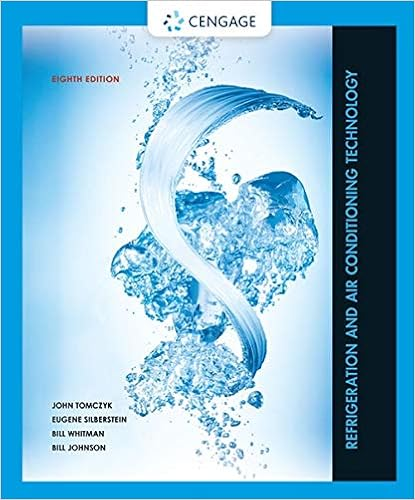# What is the next number in the sequence 6 12 24 48 a

• Test Prep
• 70

This preview shows page 9 - 12 out of 70 pages.

##### We have textbook solutions for you!
The document you are viewing contains questions related to this textbook.The document you are viewing contains questions related to this textbook.
Chapter 35 / Exercise 13
Refrigeration and Air Conditioning Technology
Silberstein/TomczykExpert Verified
7. What is the next number in the sequence? A. 72 B. 96 C. 108 D. 112 E. 124 8. Which of the following numbers could be described in the following way: an integer that is a natural, rational and whole number? B. 1 C. 2.33 D. -3 E. none of the above 9. What is the next number in the following pattern? 1, 1/2, 1/4, 1/8, ___ A. 1/10 B. 1/12 C. 1/14 D. 1/15 E. 1/16 10. Of the following units, which would be most likely to measure the amount of sugar needed in a recipe for 2 dozen cookies C. quarts D. kilograms E. cups 1. C 2. E 3. C 4. D Answer Key 5. C 6. C 7. B 8. B 9. E 10. E
##### We have textbook solutions for you!
The document you are viewing contains questions related to this textbook.The document you are viewing contains questions related to this textbook.
Chapter 35 / Exercise 13
Refrigeration and Air Conditioning Technology
Silberstein/TomczykExpert Verified
1. 10 4 is not equal to which of the following? 2. Multiply 10 4 by 10 2 A. 10 8 B. 10 2 C. 10 6 D. 10 -2 E. 10 3 3. Divide x 5 by x 2 A. x 7 B. x 4 C. x 10 D. x 3 E. x 2.5 4. Find 8.23 x 10 9 A. 0.00000000823 B. 0.000000823 C. 8.23 D. 8230000000 E. 823000000000 5. 83,000 equals: A. 83.0 x 10 4 B. 8.3 x 10 4 C. 8.3 x 10 3 D. 83.0 x 10 5 E. 83.0 x 10 2 6. .00875 equals: A. 8.75 x 10 -2 B. 8.75 x 10 -3 C. 8.75 x 10 -4 D. 87.5 x 10 -3 E. 875 x 10 -4 Answer Key
10
11 1. What is the improper fraction or mixed number represented by the following figure? 2. Which of the following fractions most correctly depicts the shaded area of the circle below? 3. Which of the following is not a fraction equivalent to 3/4? A. 6/8 B. 9/12 C. 12/18 D. 21/28 E. 27/36 4. Solve: 0.25 + 0.65 5. Which of the following statements is false? E. 33 1/3 % is equivalent to 6. Find the square of 25/9 A. 5/3 B. 3/5 C. 7 58/81 D. 15/2 E. 650/81 7. Sarah needs to make a cake and some cookies. The cake requires 3/8 cup of sugar and the cookies require 3/5 cup of sugar. Sarah has 15/16 cups of sugar. Does she have enough sugar, or how much more does she need? 8. There are 8 ounces in a 1/2 pound. How many ounces are in 7 3/4 lbs? 9. If the value of x and y in the following fraction are both tripled, how does the value of the fraction change? XZ Y A. increases by half B. decreases by half C. triples D. doubles E. remains the same
•••